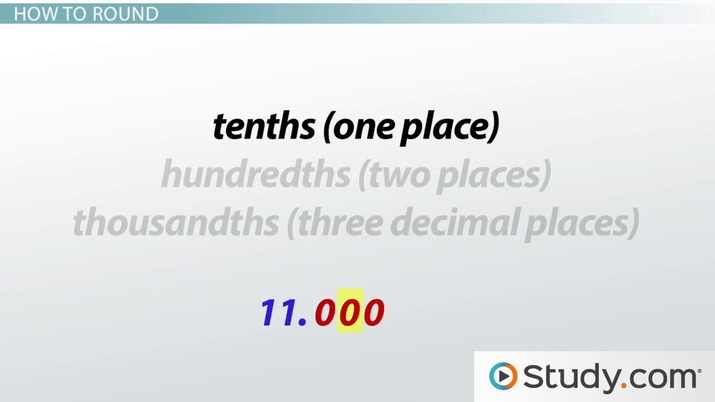# How to Round Decimals

Lesson Transcript
Instructor: Yuanxin (Amy) Yang Alcocer

Amy has a master's degree in secondary education and has been teaching math for over 9 years. Amy has worked with students at all levels from those with special needs to those that are gifted.

Decimals are numbers with a decimal point and numbers after the point. In this lesson, the reader will learn how to round decimals to any desired decimal place. Updated: 10/06/2021

## Decimals

We see decimals every day. They are your numbers with a decimal point and some numbers behind that decimal point. Think of the numbers you see when you go shopping, and you are thinking of decimal numbers. You see your favorite shoes on sale for \$45.99, and you are looking at a decimal.

Any number with a decimal point is a decimal number. The famous math number, pi, is a decimal that begins with 3.14159. When you start experimenting with different numbers on your calculator, you will see that decimal numbers happen very often. If you divide 5 by 8, you get 5/8, which equals 0.625. 8 divided by 13 is 0.6153846...An error occurred trying to load this video.

Try refreshing the page, or contact customer support.

Coming up next: Adding and Subtracting Decimals: Examples & Word Problems

### You're on a roll. Keep up the good work!

Replay
Your next lesson will play in 10 seconds
• 0:02 Decimals
• 0:49 Rounding Decimals
• 1:48 How to Round
• 3:29 Examples
• 4:26 Lesson Summary
Save Save

Want to watch this again later?

Timeline
Autoplay
Autoplay
Speed Speed

## Rounding Decimals

Did you notice that the answer to 8 divided by 13 is a decimal number that continues forever, like the number pi? It's impossible to completely write out the whole decimal. So, in math, this is where rounding our decimals comes in. Rounding decimals isn't about writing our decimals all nice and round, but it is about shortening our decimals so that we only write a few digits while trying to keep the number as accurate as possible.

You lose accuracy when you round a decimal, but you get a number that is easier to use. For example, is 3.14159265359 easier to use or 3.14? Of course, the shorter one is easier to use. Yes, 3.14 is the rounded decimal number of 3.14159265359, which is just the beginning of the number pi.

## How to Round

To round decimals, we first decide how short we want our rounded decimal. For this, we need to know our number places. For example, are we rounding to the tenths (one place), hundredths (two places), or thousandths (three decimal places)? The tenths place tells us that we want to shorten our decimal so that it only has one number after the decimal point. The hundredths place is two numbers after the decimal point. The thousandths place is the third number after the decimal point.

Once we have decided how short we want our decimal, we now look at the numbers that are being cut off, the numbers to the right of our last desired digit, specifically the digit that is directly to the right of our last desired digit. If this digit is less than 5, specifically if it is 0, 1, 2, 3, or 4, then we keep our last desired digit the same and we are done rounding. But if this digit is 5 or greater than 5, specifically, if it is 5, 6, 7, 8, or 9, then we make our last desired digit go up by one.

To unlock this lesson you must be a Study.com Member.

### Register to view this lesson

Are you a student or a teacher?